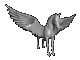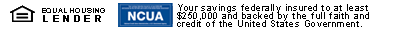Savings CalculatorThis calculator will show you interest earned and the future value of a deposit. Simply fill in the three left hand cells and then click "Compute."

Note: Your browser must support Javascript for the calculator to work!

 Dollar Amount Interest Rate Number of YearsFuture Value Interest Earned \$ \$ \$

This is for information purposes only. There may be differences in your interest calculation. This assumes a simple interest rate. For a compounded interest rate, put in the annual percentage yield (APY) in the interest rate cell.